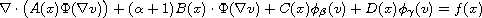Electron. J. Diff. Equ., Vol. 2011 (2011), No. 151, pp. 1-11.

### Oscillation criteria for damped quasilinear second-order elliptic equations Tadie

Abstract:
In 2010, Yoshida  stated that oscillation criteria for the superlinear-sublinear elliptic equation equationwere not known. In this article, we provide some answers to this question using boundedness conditions on the coefficients of half-linear quasilinear elliptic equations. This is obtained by using some comparison methods and Picone-type formulas.

Submitted July 21, 2011. Published November 8, 2011.
Math Subject Classifications: 34C10, 34K15, 35J70.
Key Words: Picone; oscillation criteria for half-linear elliptic equations.

Show me the PDF file (227 KB), TEX file, and other files for this article.Tadie Mathematics Institut Universitetsparken 5 2100 Copenhagen, Denmark email: tadietadie@yahoo.com, tad@math.ku.dk